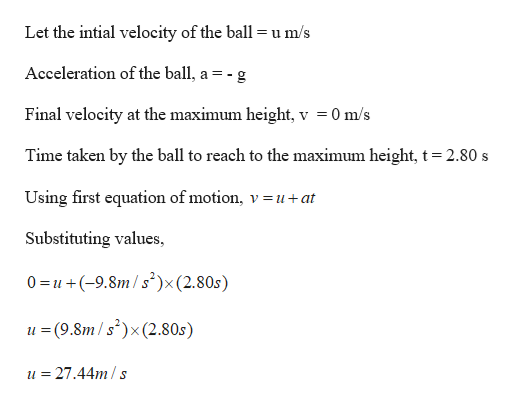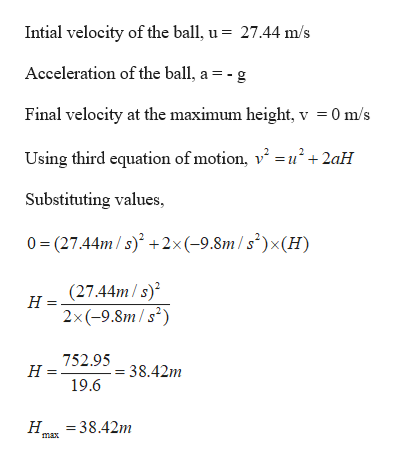# A baseball is hit so that it travels straight upward after being struck by the bat. A fan observes that it takes 2.80 s for the ball to reach its maximum height.(a) Find the ball's initial velocity.m/s upward(b) Find the height it reaches.m

Question
214 views

A baseball is hit so that it travels straight upward after being struck by the bat. A fan observes that it takes 2.80 s for the ball to reach its maximum height.

(a) Find the ball's initial velocity.
m/s upward

(b) Find the height it reaches.
m
check_circle

Step 1

Given:

Time taken by the ball to reach its maximum height, t = 2.80 s

Step 2

Calculating the initial veloctiy of the ball:help_outlineImage TranscriptioncloseLet the intial velocity of the ball u m/s = 11 Acceleration of the ball, a = - g Final velocity at the maximum height, v = 0 m/s Time taken by the ball to reach to the maximum height, t = 2.80 s Using first equation of motion, v=u + at Substituting values, 0 u (9.8m/s)x(2.80s) u (9.8m/s(2.80s) S u=27.44m/s fullscreen
Step 3

Calculating the maximum height...help_outlineImage TranscriptioncloseIntial velocity of the ball, u = 27.44 m/s Acceleration of the ball, a = - g Final velocity at the maximum height, v =0 m/s Using third equation of motion, v u+2aH Substituting values 0 (2744m/s)+2x(-9.8m/s2)x(H) H = (27.44m/s) 2x(-9.8m/s) 752.95 38.42m н 19.6 Н 338.42m max fullscreen

### Want to see the full answer?

See Solution

#### Want to see this answer and more?

Solutions are written by subject experts who are available 24/7. Questions are typically answered within 1 hour.*

See Solution
*Response times may vary by subject and question.
Tagged in

### Kinematics